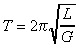Time Equations and Notes

# Time Equations and Notes

While these equations and notes are public domain general information, they do pertain to the data used in the formulae on the CSGNetwork.Com website.

 1.where T is time in seconds, l is string length in centimeters, and G is gravity. 2. solar day - 24 hours (variable). 3. sidereal day - 23 hours, 56 minutes, 4.09 seconds. 4. mean solar day - average solar day across a year - 24 hours on average. 5. second (old measure of) - 1/86,400 of the mean solar day. 6. second (present measure of) - 9,192,631,770 periods of the radiation corresponding to the transition between the two hyperfine levels of the ground state of the cesium-133 atom. 7. Coordinated Universal Time (UTC)weighted average of the various atomic time scales. 8. Ephemeris Time - is determined by the orbital motion of the Earth about the Sun (not affected by earth's rotational wobble). 9. Universal Time (UT0) - is equivalent to mean solar time at the Greenwich Meridian (Greenwich Mean Time, or GMT). 10. The Earth requires 4 minutes to bring Sun back into same point of sky every day. (Solar Day) 11. Stars set and rise on sidereal schedule. 12. Atomic time, with the unit of duration the Systeme International (SI) second defined as the duration of 9,192,631,770 cycles of radiation corresponding to the transition between two hyperfine levels of the ground state of cesium 133. 13. TAI is the International Atomic Time scale, a statistical timescale based on a large number of atomic clocks. 14. Universal time (UT) is counted from 0 hours at midnight, with unit of duration the mean solar day, defined to be as uniform as possible despite variations in the rotation of the Earth. 15. UT0 is the rotational timescale of a particular place of observation. It is observed as the diurnal motion of stars or extraterrestrial radio sources. 16. UT1 is computed by correcting UT0 for the effect of polar motion on the longitude of the observing site. It varies from uniformity because of the irregularities in the Earth's rotation. 17. Coordinated Universal Time (UTC) differs from TAI by an integral number of seconds. UTC is kept within 0.9 seconds of UT1 by the introduction of one-second steps to UTC, the "leap second" usually being a positive step. 18. Dynamical Time replaced ephemeris time as the independent argument in dynamical theories and ephemerides. Its unit of duration is based on the orbital motions of the Earth, Moon, and planets. 19. Terrestrial Time (TT), (or Terrestrial Dynamical Time, TDT), with unit of duration 86400 SI seconds on the geoid, is the independent argument of apparent geocentric ephemerides. TDT= TAI + 32.184 seconds. 20. Barycentric Dynamical Time (TDB), is the independent argument of ephemerides and dynamical theories that are referred to the solar system barycenter. TDB varies from TT only by periodic variations. 21. Geocentric Coordinate Time (TCG) is a coordinate time having its spatial origin at the center of mass of the Earth. TCG differs from TT as: TCG - TT = Lg * (JD -2443144.5) * 86400 seconds, with Lg = 6.969291e-10. 22. Barycentric Coordinate Time (TCB)is a coordinate time having its spatial origin at the solar system barycenter. TCB differs from TDB in rate. The two are related by: TCB - TDB =Lb * (JD -2443144.5) * 86400 seconds, with Lb =1.550505e-08. 23. Sidereal Time, with unit of duration the period of the Earth's rotation with respect to a point nearly fixed with respect to the stars, is the hour angle of the vernal equinox. 24. Delta T is the difference between Earth rotational time (UT1) and dynamical time (TDT). Predicted values of TDT - UT are provided by the Earth Orientation Department. 25. Julian Day Number is a count of days elapsed since Greenwich mean noon on 1 January 4713 B.C., Julian proleptic calendar. The Julian Date is, then, the Julian day number followed by the fraction of the day elapsed since the preceding noon. 26. We frequently make use of the Modified Julian Date (MJD), which is defined as MJD =JD - 2400000.5. 27. A MJD day thus begins at midnight, civil date. Julian dates can be expressed in UT, TAI, TDT, among others, and so for precise applications the timescale should be specified, for example, MJD 49135.3824 TAI. 28. one (1) mean solar day = 1.0027379093 sidereal days. 29. sidereal month - 27.3 days (one moon revolution). 30. lunar month - 29.5 days (time between full moons). 31. sidereal revolution period - revolution relative to stars. 32. synodic revolution period - oribital period as seen from earth. 33. SRP - one sidereal day. 34. SyRP - one solar day. 35. leap second - compensation in atomic clocks for earth's irregular rotational period. 36. millennium - 1000 years. 37. century - 100 years. 38. decade - 10 years. 39. leap year - 366 days. 40. year - 365 days. 41. hour - 60 minutes. 42. minute - 60 seconds. 43. millisecond - .001 seconds (1E-3). 44. microsecond - .000001 seconds (1E-6). 45. nanosecond - 1E-9 seconds. 46. picosecond - 1E-12 seconds. 47. femtosecond - 1E-15 seconds. 48. attosecond - 1E-18 seconds.